# The Gravity Force, Weight, and The Center of Gravity

0
6229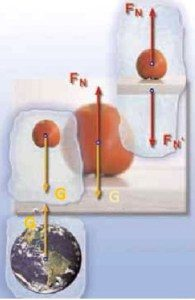When we hold an item in our hand, it presses our hand downwards. The force by which the body presses the horizontal surface on which it stands is called the weight of the body.

The gravity force and the weight of the body have the same magnitude and direction, but do not have the same center of pressure.

– The center of pressure of the gravity force that acts on the body is in the body.

– The center of pressure is at the point where the body is supported or at the point of suspension.

The weight of the body is equal to the gravity force only if the body is placed on a fixed surface or is being suspended.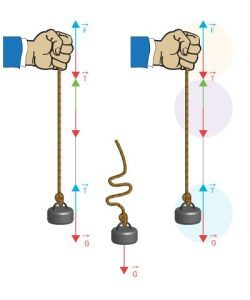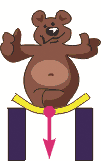The gravity force by which, due to the force of the Earth, the body presses a fixed support or tighten the thread for which they are hung is called the weight of the body. The weight of the body is equal to the product of body weight and gravitational acceleration and acts vertically downwards towards the Earth.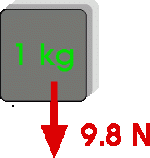In this case, the weight is equal to the Earth’s gravity force. The weight unit is same as for the force (1N). Weight of a body mass of 1kg is equal to 9.81N.

The force of gravity acts on the body, the weight acts on the surface on which the body stands or on the thread on which the body is suspended. The gravity force and the weight of the body have the same intensity and direction, but do not have the same center of the pressure. The center of the pressure of gravity is located at the point of the body called the center of gravity, while the weight of the body acts at the point of support or suspension.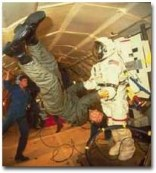If the body falls then it is in a state of zero gravity. Due to the gravity force, all  bodies that are prevented from falling are pressing down what is the supporting them from the fall or are tightening the thread that they are suspended.

Bodies everywhere in the universe, on other celestial bodies and on the Earth have the same mass.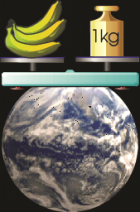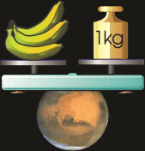The weight of the body is equal to the gravity force if the body moves uniformly.

What does the weight of the body depends on?

Physicists have determined that the body weight depends on its mass. The greater the mass of the body, the greater its weight. This means that the weight of the two bodies is equal, if their masses are equal, provided they are in the same place on the Earth’s surface where the gravitational acceleration is the same.

Example 1:

When a body mass of 1 kg its weight is 9.81N,

-When a body mass of 3kg has a weight of G = 3kg. 9.81N / kg = 29.43N; The weight is increased 3 times.

-When a body mass of 10kg has a weight of G = 10kg. 9.81N / kg = 98.1N The weight is increased 10 times.

On some other planet where the gravitational acceleration different from the acceleration of the Earth, the weight of the body of the same mass will be different. Bodies of the same mass on different planets will have different weight.

Example 2: The picture shows the body in weightless state and that the body has the same mass on the Earth and on the Moon, which is 60 kg. Although the mass is constant anywhere, the body’s weight different depending on where the body is placed. The body on the Earth has a weight of 588.6N, but the body on the Moon has a weight of 97.32 N.

The mass is constant, but for the same mass the body has almost 6 times the weight on Earth than on the Moon, because the gravitational acceleration of the Moon is gm = 1.6 m / s².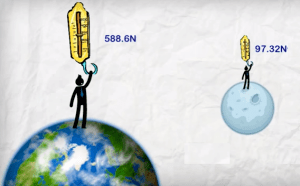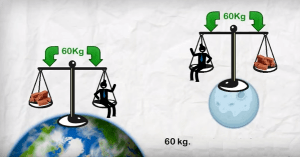The Newton’s Second law has the same form in an accelerated system as well if we add inertia to the forces created by the action of other bodies.

Example: The ratio of the weight and gravity force while using the elevator. The picture shows the weight of the fish in the elevator while the elevator moves upwards and while the elevator moves vertically downwards. In the figure a, the scale shows more weight when the lift moves upwards than the weight that the body has when the elevator is not moving or when its moving uniformly. In figure b, the scale shows less weight than the weight that the body has when the elevator is stationary or its moving uniformly.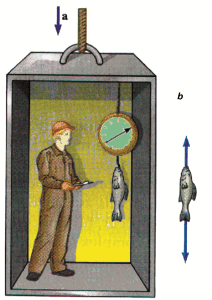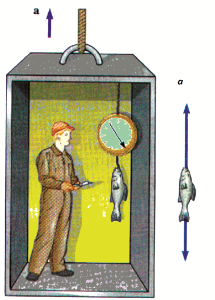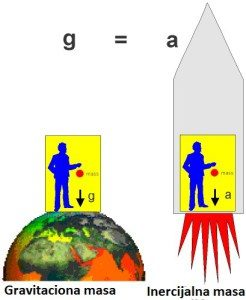From the experience we know that the body rests on a fixed base, since on the body act both the weight G and the resistance of the base FN. The magnitudes of these forces are the same and they have the same path but the opposite is the direction, so they are mutually canceled out. This force is also called the support force (reaction of the surface, the normal reaction of the surface, etc.). The vector of the support force is perpendicular to the surface, i.e. lying on the “normal” on the surface. Therefore, it is sometimes said – the normal force of the surface.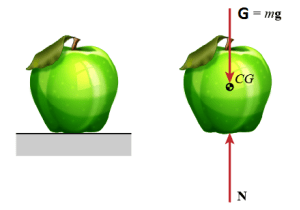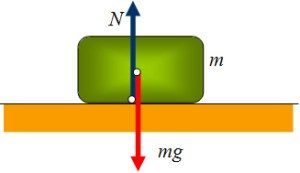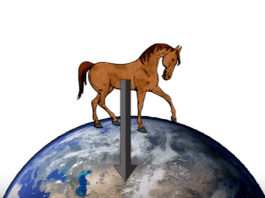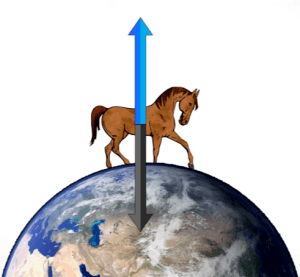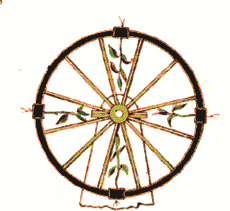Earth’s gravity is the reason why the plant’s tree grow upward, and the reason why the root goes into the ground is due to a gravitational force. If this force would have been suppressed by some stronger force, then the root of the plant would propagate in the direction of the action of that force. We can prove this in the next experiment, which English scientist Nate has done over a hundred years ago. He put the seeds on the edge of the wheel, which moves quickly. During the rapid rotation, the action of the centrifugal force surpassed the gravitational force. Since it is centered radially from the center, the edges of the plant’s root turned out, and the plant’s tree inside. This clearly shows us that the Earth’s gravity force determines the direction in which the root of the plant will be provided.

The center of gravity

Each body have a point called the center of gravity. From many examples we know that the balance of the body depends on the position of the its support on the other body, in relation to the center of gravity of the body.

Example: Move your finger to the center. At the point in which all the weight is concentrated try holding a pen. Move your finger a little to the left or to the right. What do you notice? Try holding a pen on one finger at any other point and the pen will fall.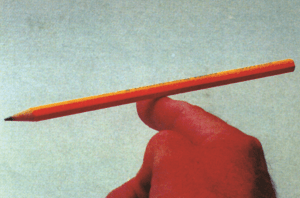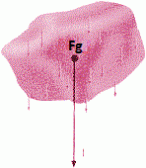What is the center of gravity?

On the body, as a system or particles, acts the force we call the resulting force of gravity acts. If each body is a sum of particles, then the resulting gravity force of the forces that acts on the body is the sum of the forces acting on each particle individually. The pressure point of that resulting gravity force is called the center of gravity. That is why all the bodies on Earth behave as if their whole weight is concentrated at one point of the center of gravity. The center of gravity is the pressure point of the resulting force of the Earth’s gravity force of all the forces that act on each particle of the body individually. How do we determine the center of gravity of the body?

The center of gravity is the pressure point of the resulting force of the Earth’s gravity force of all the forces that act on each particle of the body individually.

How do we determine the center of gravity of the body?Example 1: The figure shows a fork, a spoon, and a toothpick on the edge of the glass. The toothpick rests on a glass at the point that is its focus, which is the reason why the fork and spoon stand as seen in the picture.

The center of gravity can be easily determined for homogeneous bodies that have a proper geometric shape. The center of gravity for these bodies lies in their intersections.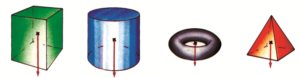If the body has no homogeneous composition, and has a proper geometric shape, its gravity center cannot be geometrically determined.Example 2: A wheel made of wood is standing on a slanted plank. That would be a paradox if the girl did not try to shift its center of gravity. She installed one metal plug near the edge of the wheel. The center of gravity of this point is very low and it is stable and at rest. Such a wheel is not a homogeneous body. And it does not have the same density of matter in all of its parts.

Example 3: We can hold a pen, a book, or any other body in one point as long as that point is the center of gravity of the body.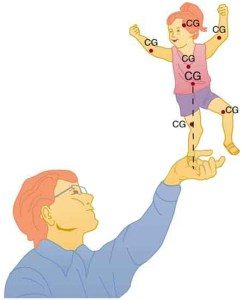Types of equilibrium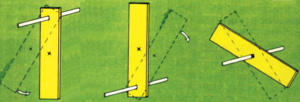According to the position of the base and the center of gravity, there are three types of balance (figure above):

– stable, the body is in a stable balance if the point of support is above the center of gravity

– unstable, the body is in a bad balance if the point of the support is below the center of gravity

– indifferent, the body is in an indifferent balance if the center of gravity is at the point of support

Stability of the body on the surface

From what depends the stability of the body on the surface?

Experience shows that the stability of the body on the surface is greater as the body weight is greater and the greater the surface of the support on which it is located. Also, the greater the center of gravity above the support is greater, the stability is lower.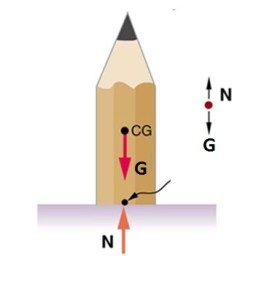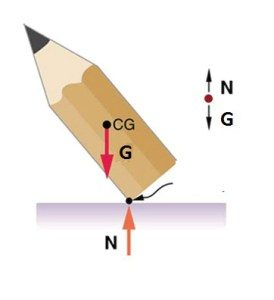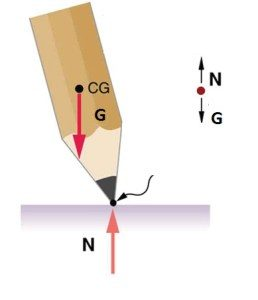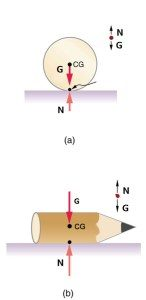Example 1: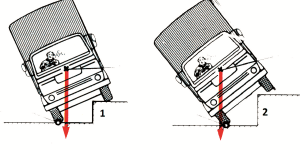The truck on the Figure 1 is stable. The normal that passing through the center of gravity is between the car wheels. The truck in Figure 2 is unstable. The normal that passing the gravity crosses the edge of the car wheels. In that case, the truck would tip over.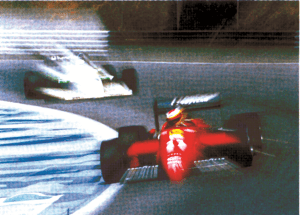“Formula 1”, on the picture, center of gravity is between the car’s wheels and it is very low near the ground. This ensures that it does not tip over during a quick ride.

Example 2: The picture shows one book in two different positions. The book is more stable in a position when a larger surface touches the surface, which is the case where the center of gravity is closer to the Earth.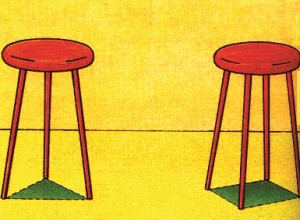The chair is more stable with four legs than three legs. The reason for that is because the larger surface is touching the surface.

Example 3: Stable and unstable chicken!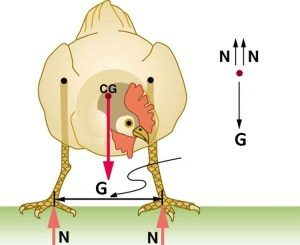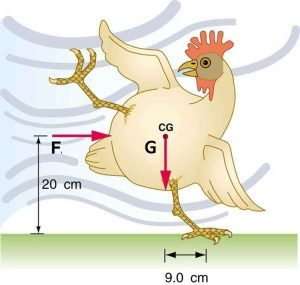From the example, we see that as the center of gravity is lower (closer to the ground) the body is more stable. For this reason, the motor in motor vehicles (cars, trucks) is set very low, and the wheels must be quite wide in order to place their center of gravities to improve the stability of the vehicle. As such it is less likely that the vehicle will tip over in the event of a collision.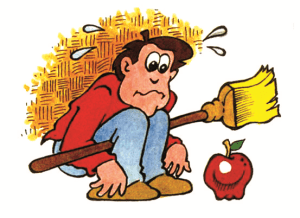SHARE
Previous articleFree Fall